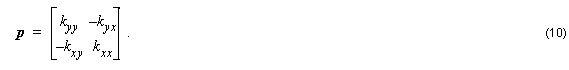## H-Formulation

The full vectorial wave equation for H is given by: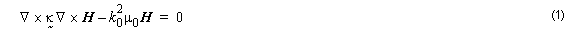By substituting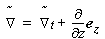into Equation 1 we get: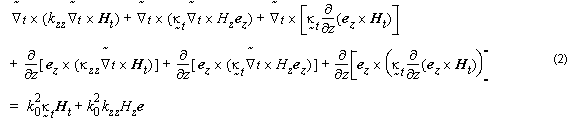The term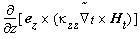is null.

We can separate Equation 2 into one for longitudinal terms and another one for transversal terms: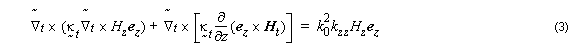for longitudinal terms, and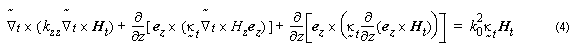By substituting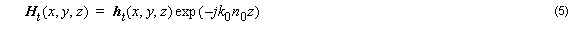into the first right hand term of Equation 4, we get: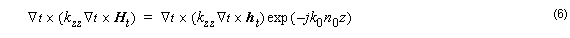By substituting Equation 5 into the second right hand term of Equation 4, we get: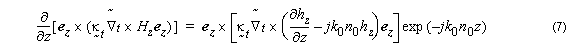The third term of Equation 4 can be rewritten as: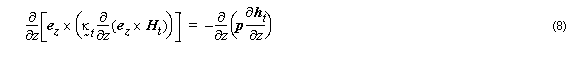By substituting Equation 5 into the second right hand term of Equation 4, we get: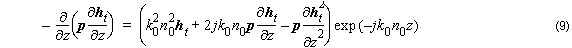where